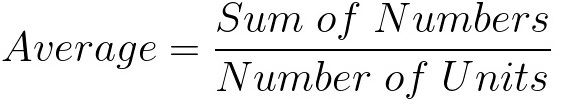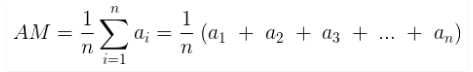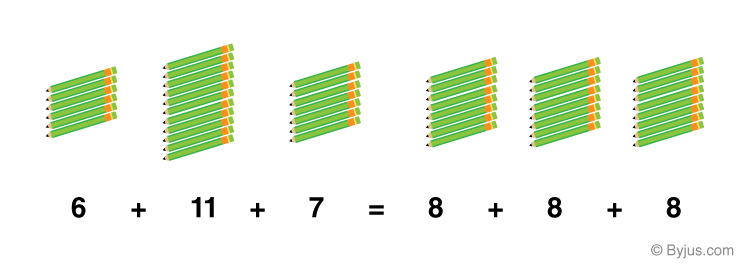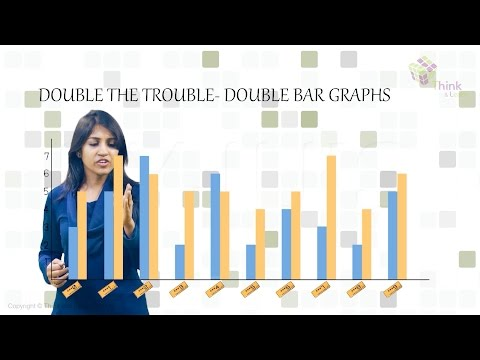# Average

In Maths, an average of a list of data is the expression of the central value of a set of data. Mathematically, it is defined as the ratio of summation of all the data to the number of units present in the list. In terms of statistics, the average of a given set of numerical data is also called mean

For example, the average of 2,3 and 4 is (2+3+4)/3 = 9/3 =3. So here 3 is the central value of 2,3 and 4.  Thus, the meaning of average is to find the mean value of a group of numbers.

 Average = Sum of Values/Number of Values

## Definition of Average

The average is defined as the mean value which is equal to the ratio of the sum of the number of a given set of values to the total number of values present in the set.

Check: Mean Definition

The average formula has many applications in real life. Suppose if we have to find the average age of men or women in a group or average male height in India, then we calculate it by adding all the values and dividing it by the number of values. Below is the formula to evaluate the average of a given set of numbers.## Symbol

The average is basically the mean of the values which are represented by x̄. It is also denoted by the symbol ‘μ’.

## Formula

The formula to find the average of given numbers or values is very easy. We just have to add all the numbers and then divide the result by the number of values given. It can be expressed as:

Average = Sum of Values/ Number of values

Suppose, we have given with n number of values such as x1, x2, x3 ,….., xn. The average or the mean of the given data will be equal to:

Average = (x1+x2+x3+…+xn)/n

## How to Calculate Average?

We can easily calculate the average for a given set of values. We just have to add all the values and divide the outcome by the number of given values. Let us understand with an example.

If there are a group of numbers say, 20, 21, 23, 22, 21, 20, 23. Then find the average of these values.

By average formula, we know,

Average = (Sum of values)/No.of values

= (20+21+23+22+21+20+23)/7

= 150/7

=21.42

### Arithmetic Mean

The Arithmetic mean is the most common type of Average. If n numbers are given, each number denoted by ai(where i = 1,2, …, n), the arithmetic mean is the sum of the as divided by n, then:where,

• n is the number of observation
• i represent the index of summation
• and ai = data value for the given index

### Geometric mean

The geometric mean is a method to find the central tendency of a set of numbers by finding the nth root of the product of n numbers. It is completely different from the arithmetic mean, where we add the observations and then divide the sum by the number of observations. But in geometric mean, we find the product of all the observations and then find the nth root of the product, provided that n is number of observations.

The formula is given by:

Geometric Mean, $$G . M=\sqrt[n]{x_{1} x_{2} \ldots x_{n}}$$

x1, x2, x3,…, xn are the individual items up to n terms

### Harmonic Mean

The harmonic mean is defined as the reciprocal of the average of the reciprocals of the given data values.

The formula to find the harmonic mean is given by:

Harmonic Mean, HM = n / [(1/x1)+(1/x2)+(1/x3)+…+(1/xn)]

Where x1, x2, x3,…, xn are the individual items up to n terms.

## Average of Negative Numbers

If there are negative numbers present in the list, then also the process or formula to find out the average is the same. Let’s understand this with an example.

Example: Find the average of 3, −7, 6, 12, −2

Solution:- The sum of these numbers

= 3 + (-7) + 6 + 12 + (-2)

= 3 – 7 + 6 + 12 – 2

= 12

Total Units = 5

Hence, average = 12/5 = 2.4

How does this whole idea of average or mean works? Average helps you to calculate on how to make all the units present in a list equal.## Solved Examples

1) Find the average of 2, 4, 6, 8.

Solution:-

Add the numbers = 2 + 4 + 6 + 8 = 20

Total Units = 4

Hence, average = 20/4 =5

2) Find the average of 6, 13, 17, 21, 23

Solution:-

= 6 + 13 + 17 + 21 + 23 = 80

Total units = 5

Hence, average = 80/5 = 16

3) If the age of 9 students in a team is 12, 13, 11, 12, 13, 12, 11, 12, 12. Then find the average age of students in the team.

Solution: Given, the age of students are 12, 13, 11, 12, 13, 12, 11, 12, 12.

Average = Sum of ages of all the students/Total number of students

A = (12+13+11+12+13+12+11+12+12)/9

A = 108/9

A = 12

Hence, the average age of students in a team is 12 years.

4) If the heights of males in a group are 5.5, 5.3, 5.7, 5.9, 6, 5.10, 5.8, 5.6, 5.4, 6. Then find the average height.

Solution: Given the height of males: 5.5, 5.3, 5.7, 5.9, 6, 5.10, 5.8, 5.6, 5.4 and 6

Average = Sum of heights of males/total number of males

A = (5.5+5.3+5.7+5.9+6+5.10+5.8+5.6+5.4+6)/10

A = 56.3/10

A = 5.63

By closely analysing these examples, one can observe that the average of a certain list of numbers is the central value of the set. Thus, the average or mean is a quantity intermediate of a set of quantities. In Mathematics, this is also called average mean.

## Video Lesson## Frequently Asked Questions – FAQs

### What is average with example?

Average is the central value of a given set of values. For example, the average of 3 and 5 is equal to (3+5)/2 = 8/2 = 4. Hence, 4 is the central value for 3 and 5.

### What is the average formula?

The formula to calculate the average of given numbers is equal to the sum of all the values divided by the total number of values.
Average = (Sum of Values/Number of Values)

### Why do we use the average formula?

Average is a way to distribute given values into equal parts. Say, the average of five values determines that the values can be divided into five equal parts. Suppose, 3,5,7,9,11 are the values.
Average = (3+5+7+9+11)/5 = 7
Thus, the sum of all the values and five times of 7 shall be equal.
3+5+7+9+11 = 35
7+7+7+7+7 = 35

### Is average and mean of numbers, same?

In simple Mathematics, the mean of values is termed as average. Therefore, logically, both average and mean is the same.

### What is the average of 10, 15, 20, 100?

Average = (10+15+20+100)/4 = 145/4 = 36.25